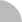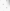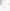# 臺灣博碩士論文加值系統

(35.172.223.30) 您好！臺灣時間：2021/07/25 12:13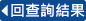:::

### 詳目顯示

: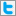Twitter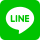•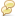被引用:0
•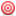點閱:226
•評分: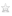•下載:20
•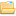書目收藏:0
 令$\mathcal{G}$表示一個圖類別,無向圖$G$是一個探測$\mathcal{G}$圖(probe $\mathcal{G}$ graph),假如我們可以在$G$中找到一個Independent Set並且在這個Independent Set中加邊,使得加完邊後的圖是一個$\mathcal{G}$圖類別中的圖,根據定義,圖類別$\mathcal{G}$是探測圖類別$\mathcal{G}$的一個子類別。給定一圖$G$，假如$G$中的任兩點在任何連通的導出子圖(induced sugraph)的距離和在$G$中的距離相同,我們稱$G$為保距圖 (distance-hereditary graph),二分保距圖(bipartite distance-hereditary graph)既是保距圖也是二分圖(bipartite graph),托勒密圖(ptolemaic graph)是弦圖(chordal graph)的子圖類別滿足圖中不含有導出子圖為gem的圖,二分保距圖和托勒密圖都是保距圖的子圖類別,在本篇論文中,我們設計了三個時間複雜度為 $O(nm)$ 的辨識演算法,用來辨識保距圖的探測圖 (probe distance-hereditary graphs),二分保距圖的探測圖 (probe bipartite distance-hereditary graphs),托勒密圖的探測圖 (probe ptolemaic graphs)。
 Let $\mathcal{G}$ denote a graph class. An undirected graph $G$ iscalled a {\em probe $\mathcal{G}$ graph} if one can make $G$ a graphin $\mathcal{G}$ by adding edges between vertices in someindependent set of $G$. By definition graph class $\mathcal{G}$ is asubclass of probe $\mathcal{G}$ graphs. A graph is {\em distancehereditary} if the distance between any two vertices remains thesame in every connected induced subgraph. {\em Bipartitedistance-hereditary graphs} are both bipartite and distancehereditary. {\it Ptolemaic graphs} are chordal and induced gem free.Both bipartite distance-hereditary graphs and ptolemaic graphs aresubclasses of distance-hereditary graphs. In this dissertation, wepropose $O(nm)$-time algorithms to recognize probedistance-hereditary graphs, probe bipartite distance-hereditarygraphs, and probe ptolemaic graphs where $n$ and $m$ are the numbersof vertices and edges of the input graph respectively.
 1 Introduction 12 Preliminaries 6 2.1 Notation 6 2.2 Properties of DHGs and its subclasses 8 2.3 Properties of probe DHGs and its subclasses 14 2.4 An $O(n^3)$-time recognition algorithm 193 Partially partitioned probe DHG 22 3.1 Twins 22 3.2 Kernel probe graphs and Algorithm B 25 3.3 Non-biconnected probe graphs without twins and Algorithm R 41 3.4 Time complexity of the main algorithm 454 Partially partitioned probe BDHG 48 4.1 False twins 49 4.2 Kernel probe graphs and Algorithm B1 51 4.3 Non-biconnected probe graphs without false twins and Algorithm R1 59 4.4 Time complexity 665 Partially partitioned PPtG 68 5.1 Some properties for reducing the problem size 69 5.2 Algorithm PPtG 87 5.3 Time complexity 946 Conclusion 97 6.1 The recognition of unpartitioned probe G graphs 97 6.2 The Hamiltonian cycle problem 98
  H. J. Bandelt, and H. M. Mulder, Distance-hereditary graphs, Journalof Combinatorial Theory, Series B 41 (1986), pp. 182–208. D. Bayer, V. B. Le, and H.N. de Ridder, Probe threshold and probetrivially perfect graphs, Theoretical Computer Science 410 (2009),pp. 4812–4822. A. Berry, E. Cohen, M. C. Golumbic, M. Lipshteyn, N. Pinet, A.Sigayret, and M. Stern, Recognition chordal-bipartite probe graphs,Research Report LIMOS/RR-07-09, 2007. A. Berry, M. C. Golumbic, and M. Lipshteyn, Recognizing ChordalProbe Graphs and Cycle-Bicolorable Graphs, SIAM Journal on Dis-crete Mathematics 21 (2007), pp. 573–591. A. Brandstädt, V. B. Le, and J. P. Spinrad, Graph classes: A sur-vey, SIAM Monographs on Discrete Mathematics and Applications,Philadelphia, 1999. D. B. Chandler, M.-S. Chang, T. Kloks, J. Liu, and S.-L. Peng, Recog-nition of probe cographs and partitioned probe distance hereditarygraphs, Proceedings of the 2nd International Conference on AlgorithmicAspects in Management (AAIM 2006), LNCS 4041 (2006), 267–278. D. B. Chandler, J. Guo, T. Kloks, and R. Niedermeier, Probe ma-trix problems: totally balanced matrices, Proceedings of the 3rd Inter-national Conference of Algorithmic Aspects on Management (AAIM2007), LNCS 4508 (2007), pp. 368–377. D. B. Chandler, M.-S. Chang, T. Kloks, J. Liu, and S.-L. Peng, Par-titioned probe comparability graphs, Theoretical Computer Science396 (2008), pp. 212–222. D. B. Chandler, M.-S. Chang, T. Kloks, V. B. Le, and S.-L. Peng,Probe ptolemaic graphs, Proceedings of the 14th Annual Interna-tional Computing and Combinatorics Conference (COCOON 2008),LNCS 5092 (2008), pp. 468–477. D. B. Chandler, M.-S. Chang, A. J.J. Kloks, J. Liu, and S.-L. Peng, Onprobe permutation graphs, Discrete Applied Mathematics 157 (2009),pp. 2611–2619. D. B. Chandler, M.-S. Chang, T. Kloks, and S.-L. Peng, Probe graphs.Manuscript, 2009http://www.cs.ccu.edu.tw/~hunglc/ProbeGraphs.pdf G. J. Chang, A. J. J. Kloks, J. Liu, and S.-L. Peng, The PIGsfull monty - a floor show of minimal separators, Proceedings of the22nd Annual Symposium on Theoretical Aspects of Computer Science(STACS 2005), LNCS 3404 (2005), pp. 521–532. G. J. Chang, T. Kloks, and S.-L. Peng, Probe interval bigraphs (ex-tended abstract), Electronic Notes in Discrete Mathematics 19 (2005),pp. 195–201. M.-S. Chang, T. Kloks, D. Kratsch, J. Liu, and S.-L. Peng, On therecognition of probe graphs of some self-complementary classes of per-fect graphs, Proceedings of the 11th Annual International Computingand Combinatorics Conference (COCOON 2005), LNCS 3595 (2005),pp. 808–817. M.-S. Chang, L.-J. Hung, and P. Rossmanith, Probe bipartite distance-hereditary graphs, Proceedings of National Computer Symposium 2009:Workshop on Algorithms and Bioinformatics, pp. 16–27. M.-S. Chang, L.-J. Hung, and P. Rossmanith, Probe distance-hereditary graphs, Proceedings of the 16th Computing: the AustralasianTheory Symposium (CATS 2010), CRPIT 109 (2010), pp. 55–64. M.-S. Chang and L.-J. Hung, Recognition of probe ptolemaicgraphs (Extended Abstract), Proceedings of International Workshopon Combinatorial Algorithms (IWOCA 2010), LNCS 6460 (2011),pp. 286–290. M.-S. Chang, L.-J. Hung, T. Kloks, and S.-L. Peng, Block-graph width,Theoretical Computer Science 412 (2011), pp. 2496–2502. M.-S. Chang, L.-J. Hung, and P. Rossmanith, Recognition of probedistance-hereditary graph graphs, accepted by Discrete Applied Math-ematics. B. Courcelle and S. Olariu, Upper bounds to the clique width of graphs,Discrete Applied Mathematics 101 (2000), pp. 77–114. B. Courcelle, J. A. Makowsky, U. Rotics, Linear time solvable optimiza-tion problems on graphs of bounded clique-width, Theory ComputingSystems 33 (2000) pp. 125–150. A. D’Atri and M. Moscarini, Distance-hereditary graphs, Steiner trees,and connected domination, SIAM Journal on Computing 17 (1988),pp. 521–538. M.C. Golumbic, H. Kaplan, and R. Shamir, Graph sandwich problems,Journal of Algorithms 19 (1995), pp. 449–473. M. C. Golumbic, M. Lipshteyn, Chordal probe graphs, Discrete AppliedMathematics 43 (2004), pp. 221–237. M. C. Golumbic, F. Maffray, and G. Morel, A characterization of chainprobe graphs, Annals of Operations Research 188 (2011), pp. 175–183. P. L. Hammer and F. Maffray, Completely separable graphs, DiscreteApplied Mathematics 27 (1990), pp. 85–99. A. Hertz, Slim graphs, Graphs and Combinatorics 5 (1989), pp. 149–157. P. Hlinˇ en´ y and S. Oum, Finding branch-decomposition and rank-decomposition, SIAM Journal on Computing 38 (2008), pp. 1012–1032. E. Howorka, A characterization of distance-hereditary graphs, TheQuarterly Journal of Mathematics 28 (1977), pp. 417–420. E. Howorka, A characterization of ptolemaic graphs, Journal of GraphTheory 5 (1981), pp. 323–331. R.-W. Hung, S. C. Wu, and M.-S. Chang, Hamiltonian cycle problemon distance-hereditary graphs, Journal of Information Science and En-gineering 19, pp. 827–838. L.-J. Hung, T. Kloks, and C. M. Lee, Trivially-perfect width, inProceedings of International Workshop on Combinatorial Algorithms(IWOCA 2009), LNCS 5874 (2009), pp. 301–311. J. L. Johnson and J. Spinrad, A polynomial-time recognition algorithmfor probe interval graphs, Proceedings of the 12th Annual ACM-SIAMSymposium on Discrete Algorithms (SODA 2001), pp. 477–486. J.-M. Lanlignel and E. Thierry, Pruning graphs with digital searchtrees. Application to distance hereditary graphs, Proceedings of the17th Annual Symposium on Theoretical Aspects of Computer Science(STACS 2000), LNCS 1770 (2000), pp. 529–541. V. B. Le and H. N. de Ridder, Characterisations and linear-time recog-nition of probe cographs. Proceedings of the 33rd International Work-shop on Graph-Theoretic Concepts in Computer Science (WG 2007),LNCS 4769 (2007), pp. 226–237. V. B. Le, Two characterization of chain partitioned probe graphs, An-nals of Operations Research 188 (2011), pp. 279–283. R. M. McConnell and J. P. Spinrad, Construction of probe intervalgraphs, Proceedings of the 13th ACM-SIAM Symposium on DiscreteAlgorithms (SODA 2002), pp. 866–875. R. M. McConnell and Y. Nussbaum, Linear-time recognition of probeinterval graphs, Proceedings of the 17th Annual European Symposiumon Algorithms (ESA 2009), LNCS 5757 (2009), pp. 349–360. S. Oum, Rank-width and vertex-minors, Journal of Combinatorial The-ory, Series B 95 (2005), pp. 79–100. S. Oum, Graphs of bounded rank-width. PhD Thesis. Princeton Univer-sity, Princeton (2005). S. Oum, Approximating rank-width and clique-width quickly, ACMTransactions on Algorithms 5, No. 1, Article 10, 2008. P. E. Zhang, A. Schon, S. G. Fischer, E. Cayanis, J. Weiss, S. Kistler,and E. Bourne, An algorithm based on graph theory for the assem-bly of contigs in physical mapping of DNA, Bioinformatics 10 (1994),pp. 309–317.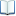電子全文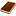國圖紙本論文推文當script無法執行時可按︰推文 網路書籤當script無法執行時可按︰網路書籤 推薦當script無法執行時可按︰推薦 評分當script無法執行時可按︰評分 引用網址當script無法執行時可按︰引用網址 轉寄當script無法執行時可按︰轉寄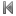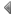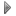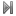top
 無相關論文

 無相關期刊

 1 最小連通支配集之演算法實作 2 最大配對導出子圖的正確解演算法 3 最密k 集合問題在最大分支度三之圖上的正確解演算法 4 最大平衡子圖之演算法 5 固定參數演算法與性質測試之研究 6 標籤不均衡資料與學習有效分類器之樣本挑選法 7 基於區域確定性的群集設計主動學習樣本資料挑選的方法 8 電子商務搜尋引擎的設計與實作 9 街景清道夫:自Google街景圖中偵測與去除車輛 10 藏匿機密資訊於影像壓縮碼及影像保護技術 11 Color CENTRIST：用於場景分類的顏色描述子 12 在有號完全圖上尋找大的平衡子集之演算法 13 視網膜血管樹重建 14 基於鏈結與相似度對網路化資料分群的主動式學習 15 YouTube 影片分類統計瀏覽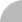簡易查詢 | 進階查詢 | 熱門排行 | 我的研究室